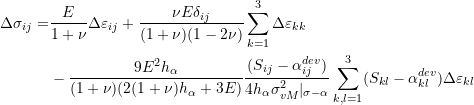### Plasticity: FEA Implementation

### Implementation in FEA:

In order to implement the nonlinear material models of plasticity described before in a finite element analysis algorithm, the overall stiffness describing the rate of change of the stress increments with respect to the strain increments is required. In particular, the components of the following fourth order tensor are required for implementation in a Newton Raphson method: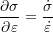whereis the stress tensor andis the total strain tensor. In order to find the components of this fourth order tensor, the incremental form of the stress-strain relationships in the Mathematical Modelling of Plasticity section are utilized. This is done for both models, the isotropic hardening model and the combined isotropic and kinematic hardening model. Note that in the derivations in the coming section, replacing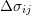with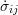and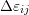with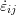will yield the same results.

### Incremental Stress-Strain Relationships for the Isotropic Hardening Model:

Adopting a vector representation of the stress and the strain increments, the increments in the stress, strain, elastic strain and plastic strain components can be represented as elements of a six dimensional vector space with the following components: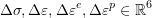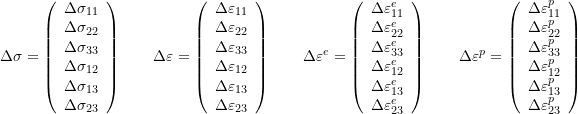When a stress increment is such that plastic strain would develop in the material, then the vector of the increments in the strain components is equal to the sum of the incremental elastic and the plastic strain components: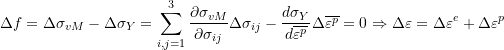Denoting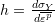, the above relationship can also be used to find an expression for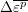: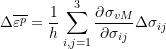The incremental elastic strain components are related to the increment in the stress components using the linear elastic constitutive behaviour: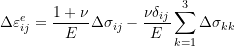which has the following matrix form: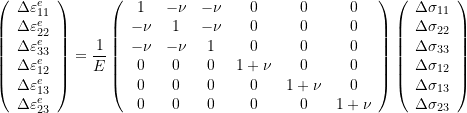The incremental plastic strain components are related to the increment in the stress components as follows after incorporating the von Mises yield function, flow rule and hardening rule: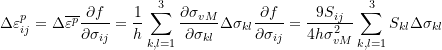Which has the following component form: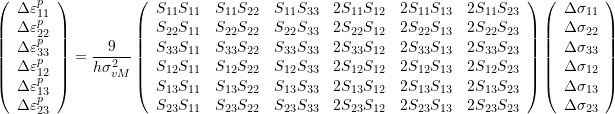where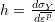is the slope of the material yield stress versus plastic strain curve and the factor 2 accounts for the symmetry of the stress tensor increments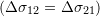. The relationships above can be written in the following form where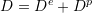represents the ivnerse of the material stiffness in the current stress increment: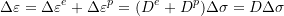In the case the case of elastic perfectly plastic materials, the value of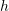is zero and the increments in the stress have to satisfy the condition that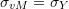where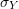is constant. In this case, the increment in the plastic strain parameteris not well defined (could be infinite) and more conditions (kinematic conditions) would be required to obtain the plastic strain components. In the case of a finite value for, the inverse relationship is required for implementation in the Newton Raphson method (i.e., the inverse of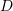). The inverse can be obtained numerically after constructing the matrices or explicitly as follows. First, the increments in the total strain components can be written in the following form: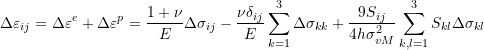In order to find the inverse of the above relationship, we first start by rearranging as follows:

(1)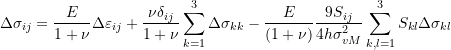In the following steps, we wish to replace the components of the stress increment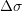in the right hand side with the components of the strain increment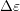. To achieve this, first, we note that if the deviatoric stress tensor components are multiplied by the increments of the total strain components and then the resulting terms are summed (i.e., obtaining the inner product of the deviatoric stress tensor and the increment in the total strain tensor), then the following expressions are obtained: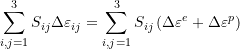The first term with the deviatoric stress tensor components multiplied by the incremental elastic strain components can be further manipulated as follows: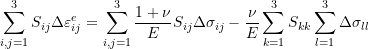The second term equals to zero since the trace of the deviatoric stress tensor is equal to zero.
On the other hand, the term with the deviatoric stress tensor components multiplied by the incremental plastic strain components can be further manipulated as follows: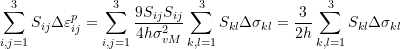Therefore:Substituting back into the third term of equation 1 results in: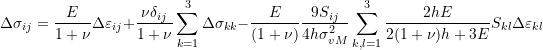Finally, the middle term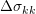can be replaced knowing that the incremental volumetric total strain is equal to the incremental volumetric elastic strain since the incremental volumetric plastic strain is equal to zero: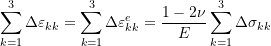Therefore, the final incremental stress total strain relationship has the following form: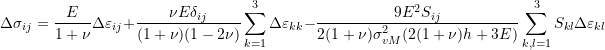The shear modulus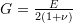can be used to further simplify the relationship as follows.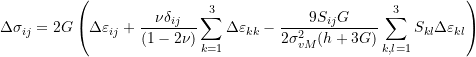Notice that this relationship can still be utilized to find the increments in the stress components if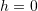(elastic perfectly plastic materials). In this case, if the increments in the total strain components are known, the associated increments in the stress components can be obtained. These increments in the stress components will ensure that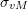does not increase since in elastic perfectly plastic material,is constant. The details of this argument are shown below. When, we have: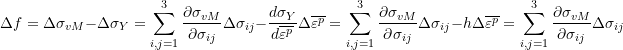By substituting forin the above equation we reach that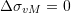as follows: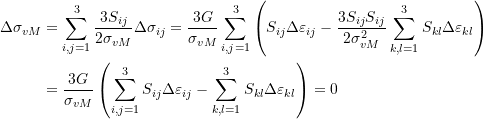### Incremental Stress-Strain Relationships for the Combined Kinematic and Isotropic Hardening Model:

We first recall the relationships for this material model:

(2)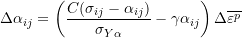where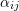are the components of the backstress tensor.

The incremental form of the consistency condition is:With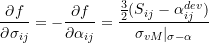Denoting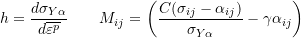and by substituting for the components of the backstress tensor in the consistency condition equation using Equation 2 we obtain: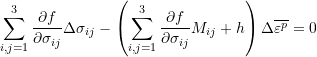Denoting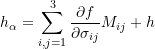then, the consistency condition is simplified to the following form: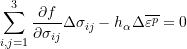The increments in the plastic strain tensor components can now be written as: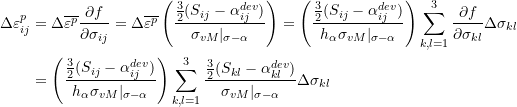Using the elastic stress strain relationships shown in the previous section and substituting the above equation in the equation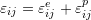yields the following relationship between the increments in the total strain and those of the stress: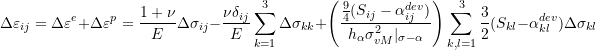Rearranging:

(3)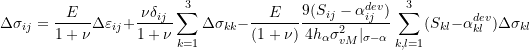Similar to the derivation for the above section, the inner production between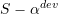andis obtained as follows: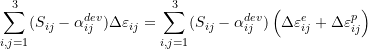The first term can be further manipulated as follows: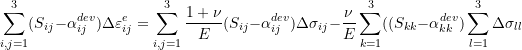The second term equals to zero since the trace ofis equal to zero.
On the other hand, the term with the deviatoric stress tensor components multiplied by the incremental plastic strain components can be further manipulated as follows: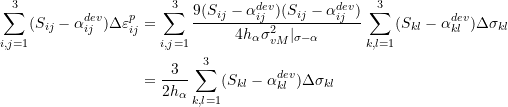Therefore: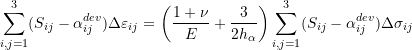Substituting back into the third term of equation 3 results in: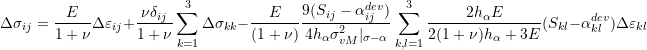Similar to the derivation in the previous section,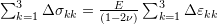which can be used to reach the final relationship: